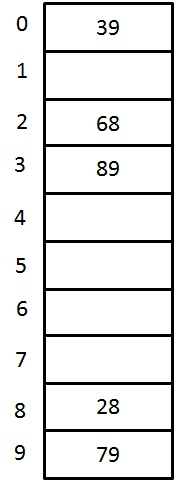# Hashing Collision avoidance - Quadratic Probing

### <<Previous - Linear Probing Next - Double Hashing >>

#### Quadratic Probing

Quadratic probing is an open addressing method for resolving collision in the hash table. This method is used to eliminate the primary clustering problem of linear probing. This technique works by considering of original hash index and adding successive value of an arbitrary quadratic polynomial until the empty location is found. In linear probing, we would use H+0, H+1, H+2, H+3,.....H+K hash function sequence. Instead of using this sequence, the quadratic probing would use the another sequence is that H+12, H+22, H+32,....H+K2. Therefore, the hash function for quadratic probing is
hi(X) = ( Hash(X) + F(i)2) % TableSize for i = 0, 1, 2, 3,...etc.
Let us examine the same example that is given in linear probing:

Solution:Fig 4. A closed Hash Table using Quadratic Probing
KeyHash Function h(X)IndexCollisionAlt Index
79h0(79)
= ( Hash(79) + F(0)2) % 10

= ((79 % 10) + 0) % 10

9
28h0(28)
= ( Hash(28) + F(0)2) % 10

= ((28 % 10) + 0) % 10

8
39h0(39)
= ( Hash(39) + F(0)2) % 10

= ((39 % 10) + 0) % 10

9The first
collision
occurs
h1(39)
= ( Hash(39) + F(1)2) % 10

= ((39 % 10) + 1) % 10

00
68h0(68)
= ( Hash(68) + F(0)2) % 10

= ((68 % 10) + 0) % 10

8The
collision
occurs
h1(68)
= ( Hash(68) + F(1)2) % 10

= ((68 % 10) + 1) % 10

9Again
collision
occurs
h2(68)
= ( Hash(68) + F(2)2) % 10

= ((68 % 10) + 4) % 10

22
89h0(89)
= ( Hash(89) + F(0)2) % 10

= ((89 % 10) + 0) % 10

9The
collision
occurs
h1(89)
= ( Hash(89) + F(1)2) % 10

= ((89 % 10) + 1) % 10

0Again
collision
occurs
h2(89)
= ( Hash(89) + F(2)2) % 10

= ((89 % 10) + 4) % 10

33

Although, the quadratic probing eliminates the primary clustering, it still has the problem. When two keys hash to the same location, they will probe to the same alternative location. This may cause secondary clustering. In order to avoid this secondary clustering, double hashing method is created where we use extra multiplications and divisions

# Hashing Collision avoidance - Quadratic Probing

### <<Previous - Linear Probing Next - Double Hashing >>

#### Quadratic Probing

Quadratic probing is an open addressing method for resolving collision in the hash table. This method is used to eliminate the primary clustering problem of linear probing. This technique works by considering of original hash index and adding successive value of an arbitrary quadratic polynomial until the empty location is found. In linear probing, we would use H+0, H+1, H+2, H+3,.....H+K hash function sequence. Instead of using this sequence, the quadratic probing would use the another sequence is that H+12, H+22, H+32,....H+K2. Therefore, the hash function for quadratic probing is
hi(X) = ( Hash(X) + F(i)2) % TableSize for i = 0, 1, 2, 3,...etc.
Let us examine the same example that is given in linear probing:

Solution:Fig 4. A closed Hash Table using Quadratic Probing
KeyHash Function h(X)IndexCollisionAlt Index
79h0(79)
= ( Hash(79) + F(0)2) % 10

= ((79 % 10) + 0) % 10

9
28h0(28)
= ( Hash(28) + F(0)2) % 10

= ((28 % 10) + 0) % 10

8
39h0(39)
= ( Hash(39) + F(0)2) % 10

= ((39 % 10) + 0) % 10

9The first
collision
occurs
h1(39)
= ( Hash(39) + F(1)2) % 10

= ((39 % 10) + 1) % 10

00
68h0(68)
= ( Hash(68) + F(0)2) % 10

= ((68 % 10) + 0) % 10

8The
collision
occurs
h1(68)
= ( Hash(68) + F(1)2) % 10

= ((68 % 10) + 1) % 10

9Again
collision
occurs
h2(68)
= ( Hash(68) + F(2)2) % 10

= ((68 % 10) + 4) % 10

22
89h0(89)
= ( Hash(89) + F(0)2) % 10

= ((89 % 10) + 0) % 10

9The
collision
occurs
h1(89)
= ( Hash(89) + F(1)2) % 10

= ((89 % 10) + 1) % 10

0Again
collision
occurs
h2(89)
= ( Hash(89) + F(2)2) % 10

= ((89 % 10) + 4) % 10

33

Although, the quadratic probing eliminates the primary clustering, it still has the problem. When two keys hash to the same location, they will probe to the same alternative location. This may cause secondary clustering. In order to avoid this secondary clustering, double hashing method is created where we use extra multiplications and divisions